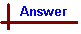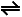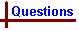Acids and Bases 2 - Questions Link to Data Page Your feedback on these self-help problems is appreciated. Click here to send an e-mail. For on-line help on this topic, please see the following Chemcal modules: Acids and Bases (strong acids and bases; conjugate acid/base pairs; pH;self-dissociation of water) Weak Acids and Bases (weak acids; weak bases; Ka and Kb) Calculations with Weak Acids and Bases (calculations involving weak acids and bases and buffers) Acid-base Titrations (titration curves for acid/base titrations; indicators) Shortcut to Questions Q: 1 2 3 4 5 6 7 8 9 10 11 1 Write an equation for the acid/base reaction in water solution between equimolar amounts of each of the following pairs and, using ionization constants, predict in each case whether reactants or products predominate at equilibrium (1.) HSO4-, SO32- (2.) H2S, H2BO3- (3.) CH3COOH, ClCH2CO2- (4.) CO32-, H2CO3 (5.) NH4+, NO2- (6.) C6H5OH, HCO3- (7.) C6H5COOH, HCO3- (8.) Fe(OH2)63+, F- (9.) N2H5+, CN- (10.) HCO3-, HPO42-2 Express the following H3O+ concentrations in terms of pH: (1.) 1.0 x 10-3 M (2.) 5.4 x 10-9 M3 Calculate [H3O+] for a solution of pH 8.37.4 Calculate the pH of a 0.10 M water solution of propanoic acid (CH3CH2COOH)5 Calculate the pH of a 0.040 M aqueous solution of hydrogen cyanide6 Calculate the pH of a 0.50 M water solution of ethylamine (CH3CH2NH2)7 At 273 K the ionic product constant of water (Kw) is 1.15 x 10-15 M2. (1.) Calculate the hydrogen ion concentration of a neutral solution at that temperature. (2.) Calculate pOH and [OH-] for a solution of pH 4.07 at 273 K.8 A particular water solution of formic acid is 3.2% ionised at 25 ° C. What is the pH of the solution?9 What is the pH of a 1.0 M solution of ethanol in water?10 Sodium (0.010 mole) is added to water (2.0 litre) (1.) What is the pH of the resulting solution? (2.) What mass of gas is given off in the reaction?11 The average pH of beer is 4.40. If the acidity is regarded as due only to the presence of dissolved carbon dioxide from the fermentation process, calculate the concentration of the carbonic acid in the beer. (Acidity arising from the second dissociation of carbonic acid or from the dissociation of water is to be neglected).Acids and Bases 2 (Answers) 1 The fundamental law of acid/base reactions states: "the stronger acid and base combine to give the weaker conjugates." This applies even when both acids involved are weak, but instead of complete reaction occurring as would be the case if strong species were involved, an equilibrium mixture results and the equilibrium lies towards the side with the weaker acid and base. All the parts in Q1 involve weak species and hence the use of equilibrium arrows in the following equations. (1.) HSO4- + SO32-SO42- + HSO3- KA of HSO4- = 10-1.99 > KA of HSO3- = 10-7.20 Therefore HSO4- is the stronger acid and products will predominate. (2.) H2S + H2BO3-HS- + H3BO3 KA of H2S = 10-7.02 > KA of H3BO3= 10-9.24 Therefore H2S is the stronger acid and products will predominate (3.) CH3COOH + ClCH2CO2-CH3CO2- + ClCH2COOH KA of ClCH2COOH = 10-2.86 > KA of CH3COOH = 10-4.76 Therefore ClCH2COOH is the stronger acid and reactants will predominate (4.) CO32- + H2CO3HCO3- + HCO3 - KA of H2CO3 = 10-6.55 > KA of HCO3 - = 10-10.33 Therefore H2CO3 is the stronger acid and reactants will predominate. (Note that there was no need to consult KA data as carbonic acid is a diprotic weak acid and its conjugate base, hydrogencarbonate ion, must necessarily be weaker because it has the same structure but carries a negative charge, making it more difficult for a H+ ion to be released.) (5.) NH4+ + NO2-NH3 + HNO2 KA of HNO2 = 10-3.14 > KA of NH3 = 10-9.24 Therefore HNO2 is the stronger acid and reactants will predominate. (6.)C6H5OH + HCO3-C6H5O- + H2CO3 KA of H2CO3= 10-6.55 > KA of C6H5OH = 10-9.98 Therefore H2CO3 is the stronger acid and reactants will predominate. (7.) C6H5COOH + HCO3-C6H5CO2- + H2CO3 KA of C6H5COOH = 10-4.20 > KA of H2CO3 = 10-6.55 Therefore C6H5COOH is the stronger acid and products will predominate. (8.) Fe(OH2)63+ + F-Fe(OH2)5(OH)2+ + HF KA of Fe(OH2)63+ = 10-2.17 > KA of HF = 10-3.17 Therefore Fe(OH2)63+ is the stronger acid and products will predominate. (9.) N2H5+ + CN-N2H4+ HCN KA of N2H5+ = 10-7.94> KA of HCN = 10-9.22 Therefore N2H5+ is the stronger acid and products will predominate. (10.) HCO3- + HPO42-H2CO3 + H2PO4- In this case, all four species ae potentially acids but both the species on the products side are diprotic acids and their conjugate bases are the reactants, so as electrically neutral H2CO3 must be a stronger acid than its negatively charged conjugate, HCO3- , and single negatively charged H2PO4- must be a stronger acid than its double negatively charged conjugate, HPO42- , then the equilibrium lies to the reactants side.2 pH = -log [H3O+] (1.) pH = -log (1.0 x 10-3 M) = 3.00 (2.) pH = -log (5.4 x 10-9 M) = 8.27 Note the conversion of significant figures between concentration and pH - the number of significant figures in the concentration becomes the number of decimal places in the pH value (and vice versa), since pH is a logarithmic scale. So in the examples above, 2 significant figures of accuracy in the concentration data results in 2 decimal places of accuracy in the resultant pH values. It is usual practice to restrict the accuracy of pH values to a maximum of 2 decimal places, since measurement approximations used to calculate pH limit it's inherent accuracy.3 [H3O+] = 10(-pH). i.e. hydrogen ion concentration is found from the inverse log of the negative pH value. ∴ Given a pH of 8.37, [H3O+] = 10-8.37 = 4.3 x 10-9 M.4 To calculate pH we need to know [H3O+]. For a weak acid such as propanoic acid, which does not dissociate completely in water, [H3O+] at equilibrium does not equal the initial concentration of the acid. So [H3O+] must first be calculated: pKA of propanoic acid = 4.87 (from data sheet). KA = 10 -4.87 Equilibrium equation: CH3CH2COOH + H2OCH3CH2CO2- + H3O+. From this equation we construct the expression for KA: KA = [CH3CH2CO2-] [H3O+] / [CH3CH2COOH] It is apparent from the equilibrium equation that each acid molecule that dissociates has to form one H3O+ and one CH3CH2CO2- ion. Therefore [H3O+] = [CH3CH2CO2-] (i.e. their concentrations must be the same). The remaining variable is [CH3CH2COOH]. Since propanoic acid is weak and only a very small amount is ionised in solution, we make the assumption that the equilibrium concentration of acid equals the initial concentration of acid (this allows us to avoid creating a quadratic expression, which is relatively time consuming to solve.) So in this example, we assume that the equilibrium concentration of propanoic acid = 0.10 M. Note that the validity of this assumption can be tested after calculation but for the purposes of exam questions in this chemistry course you can assume that the assumption is always valid. The worked problems presented in most textbooks do not assume the assumption is valid, and hence include checks as part of the working. Rearranging the expression for KA: [H3O+] [CH3CH2CO2-] = KA [CH3CH2COOH] but since [H3O+] = [CH3CH2CO2-], [H3O+]2 = KA [CH3CH2COOH] Substituting values we obtain [H3O+]2 = 10 -4.87 x 0.10 = 10-5.87 M2 ∴ [H3O+] = 10-5.87/2 M = 10-2.94 M = 1.2 x 10-3 M Finally, from [H3O+] we obtain the pH: pH = -log [H3O+] = -log (1.2 x 10-3 M) = 2.92.5 HCN + H2OH3O+ + CN- We find pH from [H3O+], which is in turn calculated from KA for HCN pKA of HCN = 9.22 (from data sheet). KA = 10 -9.22 KA = [H3O+] [CN-] / [HCN] Since [H3O+] = [CN-] ∴ KA = [H3O+]2 / [HCN] We assume [HCN] at equilibrium is equal to initial [HCN] as the amount of HCN that has dissociated is negligible compared with its initial concentration. Substituting and rearranging: KA = 10 -9.22 = [H3O+]2 / 0.040 M [H3O+]2 = 10 -9.22 x 0.040 = 10 -10.62 M2 ∴ [H3O+] = 10-10.62/2 M = 10 -5.31 M pH = -log [H3O+] = -log ( 10 -5.31 ) = 5.31.6 Ethylamine, CH3CH2NH2, has the same structure as the weak base, ammonia, NH3, except that one of the H atoms has been replaced by an ethyl group, CH3CH2-, but it is still a base as the central N atom has a lone pair of electrons that can bond to an H+ donated by an acid. The equation for the equilibrium set up by ethylamine and water is: CH3CH2NH2 + H2OCH3CH2NH3+ + OH- pKA of the conjugate acid of ethylamine, the ethylammonium ion, CH3CH2NH3+ = 10.67. Therefore pKB of CH3CH2NH2 (the conjugate base of CH3CH2NH3+) = pKW - pKA(CH3CH2NH3+) = 14 - 10.67 = 3.33. ∴ KB for ethylamine= 10 -3.33 M. KB = [CH3CH2NH3+] [OH-] / [CH3CH2NH2] As there is no other source of hydroxide ions, [CH3CH2NH3+] = [OH-] ∴ KB = [OH-]2 / [CH3CH2NH2] We can take [CH3CH2NH2] at equilibrium as equal to initial [CH3CH2NH2] as the extent of hydrolysis of a weak base is negligible compared with its initial concentration. 10 -3.33 = [OH-]2 / 0.50 M [OH-]2 = 10 -3.33 x 0.50 = 10 -3.63 M2 [OH-] = 10 -3.63/2 = 10 -1.82 M pOH = -log [OH-] = 1.82 ∴ pH = 14 - pOH = 14 - 1.82 = 12.18.7 (1.) Equation for the self-ionization of water: H2O + H2OH3O+ + OH- By definition, KW = [H3O+] [OH-]. Since [H3O+] = [OH-], KW = [H3O+]2 ∴ [H3O+] = (KW)½ = (1.15 x 10-15)½ = 3.39 x 10-8 M (2.) pH = 4.07. [H+] = 10(-pH) = 10-4.07 = 8.51 x 10-5 M [OH-] = KW / [H+] = 1.15 x 10-15 / 8.51 x 10-5 = 1.35 x 10-11 M ∴ pOH = -log [OH-] = -log (1.35 x 10-11 M) = 10.87.8 The first step is to calculate the concentration of the HCOOH solution from KA and the ionisation information. HCOOH + H2OHCO2- + H3O+ pKA = 3.74 (from data sheet) KA = 10 -3.74 M = 1.84 x 10-4 M = [HCO2-] [H3O+] / [HCOOH] "3.2 % ionised" implies that there are 3.2 HCO2-and 3.2 H3O+ ions per 100 HCOOH molecules initially present. Let the initial [HCOOH] = Z M. Then [HCO2-] = [H3O+] = 0.032 x Z M, and strictly the equilibrium [HCOOH] = Z - 0.032 Z = Z(1 - 0.032) = 0.968 Z M. However, following the procedure used previously, the equilibrium [HCOOH] can be taken as Z M. Substituting this in the expression for KA : KA = [HCO2-][H3O+] / [HCOOH] = (0.032 x Z)(0.032 x Z) / Z = 1.84 x 10-4 M ∴ (0.032 x Z)(0.032) = 1.84 x 10-4 M Z = [HCOOH] = 1.84 x 10-4 / (0.032 x 0.032) = 0.18 M. Now that the concentration is known, the pH is easily determined: KA = [H3O+]2 / [HCOOH] ∴ [H3O+] = (1.84 x 10-4 x 0.18)½ = 5.75 x 10-3 M. pH = -log [H3O+] = -log (5.70 x 10-3 M) = 2.249 Ethanol acting as acid: CH3CH2OH + H2OCH3CH2O- + H3O+ Water acting as acid: H2O + H2OOH- + H3O+ pKA of CH3CH2OH is greater than 14 (from data sheet). This means that H2O (which has a pKA equal to 14) is a stronger acid than ethanol. The contribution of H3O+ from the self ionisation of water is greater than the contribution from ethanol, and the pH of the solution is determined by the water present. As a result, the pH of the ethanol solution is 7.00 - the same as if the ethanol were not present.10 Na + H2O → NaOH + ½H2 (1.) From the above equation 0.010 mole sodium will react to form 0.010 mole NaOH. Molarity = no. mol / volume = 0.010 mol / 2.0 L = 0.0050 M. NaOH is very soluble, and completely dissociates in solution, so [OH-] = 0.0050 M. [H3O+] = KW / [OH-] = 1.0 x 10-14 M2 / 0.0050 M = 2.0 x 10-12 M. ∴ pH = -log (2.0 x 10-12 M) = 11.7 (2.) Mass of H2 gas = no. mol H2 x molar mass H2 = 0.0050 mol x 2.016 g mol-1 = 0.011 g.11 pH = 4.40, ∴ [H3O+] = 10-4.40 M. H2CO3 + H2OH3O+ + HCO3- pKA of H2CO3 = 6.35. KA = [H3O+] [HCO3-] / [H2CO3] = 10-6.35 M [H3O+] = [HCO3-] ∴ [H2CO3] = [H3O+]2 / KA = (3.98 x 10-5 M)2 / 4.47 x 10-7 M = 3.5 x 10-3 M.